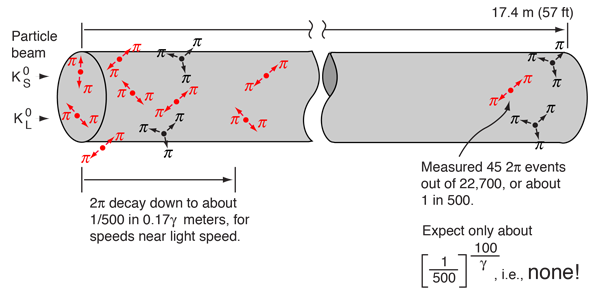# Cronin and Fitch Experiment with Kaons

In the study of neutral kaons, two particle species were found, having apparently identical masses but very different lifetimes. These particles have different decay modes.In 1964, Cronin and Fitch performed a beam experiment in which they measured the decay of pions at the end of a 57 foot beamline. Given the disparity of the lifetimes of the two kaon species, you expect to see only the long-lived version at the end of the beam tube, but they found about 1 in 500 decays to be 2-pion decays, characteristic of the short-lived species.The short-lived particle should not be observable more than a few centimeters down the beam line. To show that, suppose we calculate the time required to drop to 1/500 the original population. With the stated lifetime, the halflife calculation gives a time of 5.5 x 10-10 seconds. At nearly the speed of light, this would give a distance of about 17 centimeters, only 1/100 the length of Cronin and Fitch's beam tube. The problem with this number is that it ignores relativistic time dilation, and the lifetime as seen in the laboratory frame is dilated by the relativity factor γ (at 0.98c, γ =5). The revised distance is then 17γ cm, so even at 0.98c the population would be down by a factor of 500 by the time you got a meter down the beam tube. So for any expected particle velocity, you would expect all of them to have decayed long before you get to the end of the beam line.

This experiment showed that there was a small CP violation in the kaon decay, so the kaon has played a central role in the discussions of these symmetries since that time.

 Table of mesons Meson diagram
Index

Particle concepts

Reference
Griffiths
Ch. 4

Das & Ferbel
Ch. 12

 HyperPhysics***** Quantum Physics R Nave
Go Back

# CP Violation in Kaon Decay

The neutral kaon decay is the only experimental example of CP violation. The Cronin and Fitch experiment was the first to demonstrate it, but the decay of the kaons to pions and electrons is more clear-cut. The two processes of decay are:

K0L -> π+ + e- + ν e

K0L -> π- + e+ + ν e

These decays are called semileptonic decays, producing one meson and two leptons. They account for about 39% of K0L decays compared to 34% for the 3π mode. Using the CP operations

CP[π+ + e- + ν e ] = π- + e+ + ν e

we see that CP transforms one set of decay products into the other. CP invariance would then suggest an identical probability for the two. But the experimental evidence is that the positron decay pattern happens more often than the electron decay. The fractional excess is only 3.3 x 10-3, but this tiny excess suggests a difference between matter and antimatter. This small violation of CP invariance might hold part of the answer to why the universe consists almost entirely of matter rather than antimatter.

 Table of mesons Meson diagram Matter-antimatter problem
Index

Particle concepts

Reference
Griffiths
Sec 4.8

 HyperPhysics***** Quantum Physics R Nave
Go Back